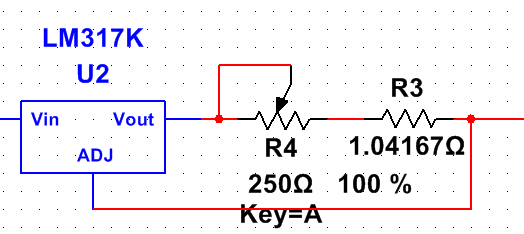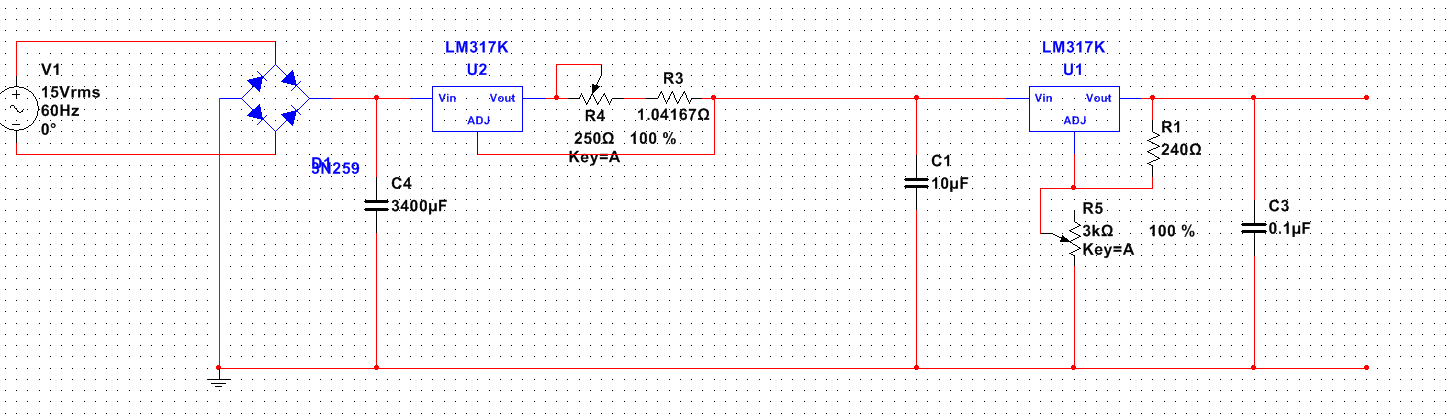# Smartest way to use current limit using LM317?

As i understand, using this circuit:Will cause the current to go right through the POT and resistor, which will generate a lot of heat. Is there a better way to use current limiting with LM317 without all of the current passing through the resistor? (Without spending a lot of money for a high power resistor/POT which can handle over 5 amps)

if anyone could draw an example circuit i would appriciate it!

I wanna be able to set a limit from 10mA to 1.2A, the output of the current limiter goes directly into another LM317 for voltage regulation. Input voltage i'm going to use is 15V.

This is how the completed project "should" look like:The output voltage is not that important for me, as long as i can get from 1.25 - 10v. I just want to avoid the heat from the current limiting part.

• Edit again and explain your requirements. Input voltage, min current, max current. Feb 4, 2016 at 22:49
• Done, sorry for the lack of info :)
– Xane
Feb 4, 2016 at 22:53
• ... and what output voltage range are you hoping for on the finished project? (You may lose too much on the too series regulators.) If you supply all the relevant details someone may come up with a better solution for you. Pop the details into the question (not in the comments). Feb 4, 2016 at 22:56
– Xane
Feb 4, 2016 at 23:02
• Your second schematic has the same problem as the first, the current will still go through r4 and r3 and u2. Feb 4, 2016 at 23:37

See LM317 based power supply with current limiting on SE and alexan_e's answer. Read through all the answers and comments as there were some good suggestions. The circuit avoids high-current pots by using some trickery.Source: ON-Semi datasheet.

Other circuits from the web:simulate this circuit – Schematic created using CircuitLab

Figure 1. Negative rail supply for first option.

Since you have plenty of transformers you can use a second one to generate the negative supply. A 6 to 9 V transformer would do the trick and I don't think you would need a regulator. A 100 mA rating would be plenty.

Understanding the constant-current sourceFigure 2. Constant current portion of the LM317 based power supply with current limiting.

As you determined in your OP, there's a problem using a variable resistor in a current source mode because all the current goes through the variable resistor. This circuit addresses that problem.

• $R_{SC}$ is a fixed resistor and sets the short-circuit (SC) current limit. The LM317 Vout is $V_{ADJ} + 1.25$ so if we want 2 A max then 0.65 Ω would do the trick.
• Q1 forms a simple constant current generator - I don't know what current it will settle down at. Maybe someone can enlighten us in the comments but let's assume it's around 10 mA going to the -10 V rail.
• D1 and D2 form a little constant-voltage drop. Whatever the voltage is on the right of $R_{SC}$ ($V_{IN2}$) the bottom of D2 will be 1.4 V (2 x 0.7 V diode drops) below $V_{IN2}$.
• By adding the 1k pot across the diodes we can adjust the voltage on $V_{ADJ}$ from Vin2 to 1.4 V below $V_{IN2}$. Remember that we only need to get $V_{ADJ}$ 1.25 V below Vout1 to shut the output off.

Time for some calculations:

$R_{sc}$ = 0.65 A. Pot wiper at top. $V_{OUT}$ feeding a low resistance load to make sure we're in current limit.

• $V_{OUT1}$ will increase until the voltage across $R_{SC}$ is 1.25 V. This will happen at 2 A.

Pot wiper now moved to centre.

• Because of the Q1, D1, D2 arrangement the voltage on the pot wiper will be Vin2 - 0.7 V.
• The output will settle when the voltage drop across $R_{SC}$ = 1.25 V - the 0.7 V = 0.55 V. From Ohm's Law, $I_{RSC} = \frac {V_{RSC}}{R_{SC}} = \frac {0.55}{0.65} = 0.85~A$.
• The further down we adjust the wiper the more the current is limited. Note that in this arrangement the current will be zero before the end of travel of the pot. Adding a series resistor at the bottom between it and the D2 junction will fix this.simulate this circuit

Figure 3. Using full span of pot by addition of R1.

• The voltage across D1 and D2 is 1.4 V, as discussed above.
• With wiper at top we get maximum current, as discussed above.
• To use the full span of the pot we want 1.25 V on the wiper when it is a bottom. Without R1 we'll have 1.4 V. $\frac {1.25}{1.4} = 0.89 = 89\%$. So we need the 1 kΩ pot to be 89% of R1 + R2. We can get this if we set $R_{TOTAL} = \frac {1k}{89} \cdot 100 = 1,123~Ω$. So R1 = 120 Ω should do the trick.

Calculating $R_{SC}$

The LM317 can handle 1.5 A max. That's your short-circuit current. $R_{SC} = \frac {V_{ADJ}}{I_{MAX}} = \frac {1.25}{1.5} = 0.83 Ω$. 0.82 Ω will do or some parallel combination.

The power rating of the resistor is given by $P = V \cdot I = 1.25 \cdot 1.5 = 1.9~W$.

• Thank you for the info, I have checked in the datasheet of LM317 and found the "Lab Power Supply Circuit", the problem with that circuit is that it needs one rail with negative voltage, i have checked a thread here about a guy who asked what was the best way to get negative voltage and he was using a center tap transformer which i don't have. I don't know how to get negative voltage without a center tap/buying additional IC.s. I do have a lot of transformers laying around but no one with a center tap.
– Xane
Feb 5, 2016 at 18:58
• See the update. Feb 5, 2016 at 19:21
• Thank you very much, I've been looking for this. Gonna try it out in a simulation :)
– Xane
Feb 5, 2016 at 20:00
• i.stack.imgur.com/oLuPR.jpg quick question, after Vout1 there is a resistor called "RSC" do you know what that stands for? Thanks!
– Xane
Feb 5, 2016 at 20:15
• Also, won't the current go right through the RSC resistor?
– Xane
Feb 5, 2016 at 20:34

You have problems and you need to listen-up. Your lower circuit shows a 15V RMS supply that is bridge rectified and smoothed. This produces a nominal DC level of about 20V. From your question you may want to push out 1.25V at 1.2A.

From this alone i can tell you that the heat dissipation in the LM317(s) is going to be: -

Power = volts dropped across LM317 x current = (20 - 1.25)V x 1.25A = 23.4 watts.

I just want to avoid the heat from the current limiting part.

The only way you can avoid this is by using a switching regulator that is configured to produce a constant current. Yes it will be noisy and yes you can make a basic switching circuit quieter but how far do you want to go?

• Yes iam aware that teh LM317 is going to get HOT. I'm gonna be cooling it using a water cooled heat sink. But that is not a problem for me, I really wanted to do this with just an LM317. But then i will start looking for another volt regulator!
– Xane
Feb 4, 2016 at 23:38
• Start looking for a buck regulator circuit that can do current limiting efficiently - there are circuits about via google. Also be aware that you ought to consider "accepting" answers to previous questions you've raised that have useful answers. Call it pay-back time for all the great and useful info you get free of charge!! Feb 4, 2016 at 23:39
• Much worse than the regulator heat is finding a good pot (not a rheostat) which will handle 1.2 amps through the wiper. Feb 5, 2016 at 4:06
• @WhatRoughBeast I don't disagree but as the op asked for heat from the current limiting part I explained that it could be as high as 23 watts. I regard the LM317 as at the heart of "the current limiting part". Feb 5, 2016 at 9:29
• You can't use a linear regulator as Andy aka told you. You need a switching regulator that limits the current to 2A and outputs 1.25V and 10mA at 10V. You need a current sensing circuit to sense the current and adjust the bias voltage at the feedback pin of the switcher. I've done this kind of circuit and it works pretty well. Feb 5, 2016 at 21:27

LM338 solves the 3 A requirement. It is good for 5 A, if used with an appropriate heatsink. This is the largest linear regulator in current production, that is normally available.

It is possible to parallel LM338 even x 3, if you know what you are doing. The National Semiconductor example shows only a master and one in parallel, but if you add extra op amps and half the sense resistor, you can go for three or even four with the original master regulator.